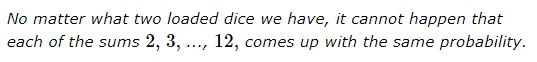### Problem### Solution 1

Let $p_i$ be the probability of $i,$ $i=1,\ldots,6,$ coming up for the first die and $q_i$ for the second. Assuming all the sums come up with the same probability, the latter equals $\displaystyle \frac{1}{11}.$ Then $p_{\small{1}}q_{\small{1}}$ is the probability of the sum being $2$ such that $p_{\small{1}}q_{\small{1}}=\displaystyle \frac{1}{11}.$ Similarly, $p_{\small{6}}q_{\small{6}}=\displaystyle \frac{1}{11}$ is the probability of the sum being $12.$

The probability of the sum being $7$ is

\displaystyle\begin{align} \frac{1}{11}&=\sum_{k+j=7}p_{\small{k}}q_{\small{j}}\gt p_{\small{1}}q_{\small{6}}+p_{\small{6}}q_{\small{1}}\\ &=\frac{p_{\small{1}}}{11p_{\small{6}}}+\frac{p_{\small{6}}}{11p_{\small{1}}}=\frac{1}{11}\left(\frac{p_{\small{1}}}{p_{\small{6}}}+\frac{p_{\small{6}}}{p_{\small{1}}}\right)\ge\frac{1}{11}\cdot 2, \end{align}

by the AM-GM inequality. That's a contradiction.

### Solution 2

Let $\displaystyle P(x)=\sum_{k=1}^6p_ix^{i-1}$ be a probability generating function for the first die, $\displaystyle Q(x)=\sum_{k=1}^6q_ix^{i-1}$ that for the second. Since $p_p,q_p\ne 0,$ each of $P(x),Q(x)$ is a polynomial of degree $5$ and, as such, has a real root.

On the other hand,

$\displaystyle P(x)Q(x)=\sum_{k=2}^{12}\frac{1}{11}x^{k-2}=\frac{1}{11}\cdot\frac{x^{11}-1}{x-1}.$

The contradiction comes from the fact that the cyclotomic polynomial $\displaystyle \sum_{k=0}^{10}x^{k}$ does not have real roots.

### Solution 3

Let $X$ denote the sum of the two dice. Suppose, for contradiction, that there exists some $p \in (0,1)$ such that

$p = P(X=2) = P(X=3) = \cdots = P(X=12).$

On the one hand, we have

$1 = P( 2 \leq X \leq 12) = P(X=2) + P(X=3) + \cdots + P(X=12) = 11p,$

which yields $\displaystyle p = \frac{1}{11}.$

On the other hand, for a fixed $x \in \{2,3, \dots, 12 \}$, we may write

\displaystyle \begin{align} p &= P(X = x)\\ &= \small{\frac{\# \text{ of elements in the sample space such that the sum of the two dice is } x} {\text{total } \# \text{ of elements in the sample space}}}\\ &= \frac{\text{a positive integer}}{36}. \end{align}

We have established that

$\displaystyle \frac{1}{11} = \frac{\text{a positive integer}}{36} \quad \Rightarrow \quad 36 = 11 \times (\text{a positive integer}),$

which is absurd.

### Generalization

The first two solutions are automatically extended to the dice of different shapes, e.g., all Platonic solids. However, the second solution would not work for a polyhedron with an odd number of faces, e.g., for a cube with a corner sawed off.

### Acknowledgment

The problem and the first two solutions come from

B. Bollobás, The Art of Mathematics: Coffee Time in Memphis, Cambridge U Press, 2006, Problem 11.

Warning: one solution is incorrect.

This problem has a very unexpeced follow-up.